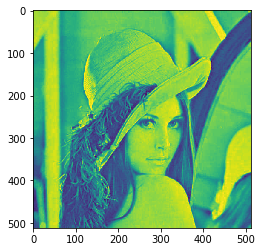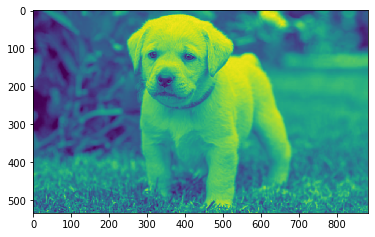GFG App
Open AppBrowser
Continue

# Mahotas – Appropriate structuring element of image

In this article we will see how we can get the appropriate structuring element of the image in mahotas. A structuring element is a matrix that identifies the pixel in the image being processed and defines the neighborhood used in the processing of each pixel.
In this tutorial we will use “lena” image, below is the command to load it.

`mahotas.demos.load('lena')`

Below is the lena imageIn order to do this we will use mahotas.get_structuring_elem method
Syntax : mahotas.get_structuring_elem(img, n)
Argument : It takes image object and integer as argument
Return : It returns numpy ndarray

Note : Input image should be filtered or should be loaded as grey
In order to filter the image we will take the image object which is numpy.ndarray and filter it with the help of indexing, below is the command to do this

`image = image[:, :, 0]`

Below is the implementation

## Python3

 `# importing required libraries` `import` `mahotas` `import` `mahotas.demos` `from` `pylab ``import` `gray, imshow, show` `import` `numpy as np` `import` `matplotlib.pyplot as plt` `  `  `# loading image` `img ``=` `mahotas.demos.load(``'lena'``)`     `  `  `# filtering image` `img ``=` `img.``max``(``2``)`   `print``("Image")` `  `  `# showing image` `imshow(img)` `show()`   `# getting structuring element` `value ``=` `mahotas.get_structuring_elem(img, ``1``)` ` `  `# showing value` `print``(value)`

Output :

`Image````[[0 1 0]
[1 1 1]
[0 1 0]]```

Another example

## Python3

 `# importing required libraries` `import` `mahotas` `import` `numpy as np` `from` `pylab ``import` `gray, imshow, show` `import` `os` `import` `matplotlib.pyplot as plt` ` `  `# loading image` `img ``=` `mahotas.imread(``'dog_image.png'``)`     `# filtering image` `img ``=` `img[:, :, ``0``]` `  `  `print``("Image")` `  `  `# showing image` `imshow(img)` `show()`   `# getting structuring element` `value ``=` `mahotas.get_structuring_elem(img, ``2``)` ` `  `# showing value` `print``(value)`

Output :

`Image````[[1 1 1]
[1 1 1]
[1 1 1]]```

My Personal Notes arrow_drop_up